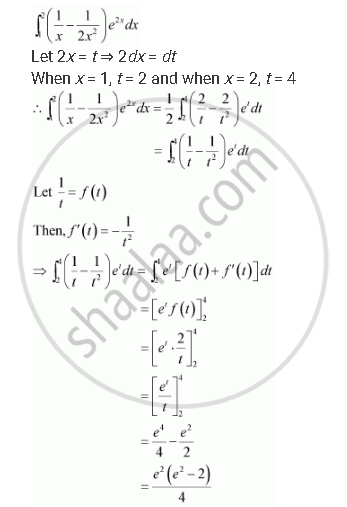Share
Notifications

View all notifications
Books Shortlist
Your shortlist is empty

# Solution for Evaluate the Integrals in Using Substitution Int_1^2 (1/X- 1/(2x^2))E^(2x) Dx - CBSE (Science) Class 12 - Mathematics

Login
Create free account

Forgot password?
ConceptEvaluation of Definite Integrals by Substitution

#### Question

Evaluate the integrals in using substitution

int_1^2 (1/x- 1/(2x^2))e^(2x) dx

#### SolutionIs there an error in this question or solution?

#### Video TutorialsVIEW ALL 

Solution Evaluate the Integrals in Using Substitution Int_1^2 (1/X- 1/(2x^2))E^(2x) Dx Concept: Evaluation of Definite Integrals by Substitution.
S